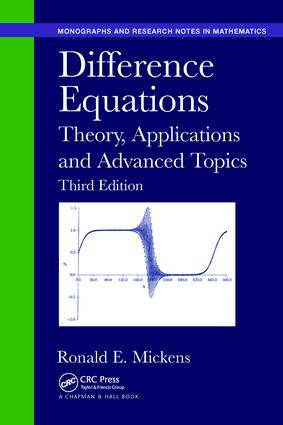Difference Equations

Theory, Applications and Advanced Topics, Third Edition, 3rd Edition

Chapman and Hall/CRC

555 pages

Purchasing Options:\$ = USD
Paperback: 9781138894235
pub: 2018-04-09
SAVE ~\$13.99
\$69.95
\$55.96
x
Hardback: 9781482230789
pub: 2015-03-24
SAVE ~\$37.00
\$185.00
\$148.00
x
eBook (VitalSource) : 9780429160455
pub: 2015-03-06
from \$34.98

FREE Standard Shipping!

Description

Difference Equations: Theory, Applications and Advanced Topics, Third Edition provides a broad introduction to the mathematics of difference equations and some of their applications. Many worked examples illustrate how to calculate both exact and approximate solutions to special classes of difference equations. Along with adding several advanced topics, this edition continues to cover general, linear, first-, second-, and n-th order difference equations; nonlinear equations that may be reduced to linear equations; and partial difference equations.

New to the Third Edition

• New chapter on special topics, including discrete Cauchy–Euler equations; gamma, beta, and digamma functions; Lambert W-function; Euler polynomials; functional equations; and exact discretizations of differential equations
• New chapter on the application of difference equations to complex problems arising in the mathematical modeling of phenomena in engineering and the natural and social sciences
• Additional problems in all chapters
• Expanded bibliography to include recently published texts related to the subject of difference equations

Suitable for self-study or as the main text for courses on difference equations, this book helps readers understand the fundamental concepts and procedures of difference equations. It uses an informal presentation style, avoiding the minutia of detailed proofs and formal explanations.

THE DIFFERENCE CALCULUS

GENESIS OF DIFFERENCE EQUATIONS

DEFINITIONS

DERIVATION OF DIFFERENCE EQUATIONS

EXISTENCE AND UNIQUENESS THEOREM

OPERATORS ∆ AND E

ELEMENTARY DIFFERENCE OPERATORS

FACTORIAL POLYNOMIALS

OPERATOR ∆−1 AND THE SUM CALCULUS

FIRST-ORDER DIFFERENCE EQUATIONS

INTRODUCTION

GENERAL LINEAR EQUATION

CONTINUED FRACTIONS

A GENERAL FIRST-ORDER EQUATION: GEOMETRICAL METHODS

A GENERAL FIRST-ORDER EQUATION: EXPANSION TECHNIQUES

LINEAR DIFFERENCE EQUATIONS

INTRODUCTION

LINEARLY INDEPENDENT FUNCTIONS

FUNDAMENTAL THEOREMS FOR HOMOGENEOUS EQUATIONS

INHOMOGENEOUS EQUATIONS

SECOND-ORDER EQUATIONS

STURM–LIOUVILLE DIFFERENCE EQUATIONS

LINEAR DIFFERENCE EQUATIONS

INTRODUCTION

HOMOGENEOUS EQUATIONS

CONSTRUCTION OF A DIFFERENCE EQUATION HAVING SPECIFIED SOLUTIONS

RELATIONSHIP BETWEEN LINEAR DIFFERENCE AND DIFFERENTIAL EQUATIONS

INHOMOGENEOUS EQUATIONS: METHOD OF UNDETERMINED COEFFICIENTS

INHOMOGENEOUS EQUATIONS: OPERATOR METHODS

z-TRANSFORM METHOD

SYSTEMS OF DIFFERENCE EQUATIONS

LINEAR PARTIAL DIFFERENCE EQUATIONS

INTRODUCTION

SYMBOLIC METHODS

LAGRANGE’S AND SEPARATION-OF-VARIABLES METHODS

LAPLACE’S METHOD

PARTICULAR SOLUTIONS

SIMULTANEOUS EQUATIONS WITH CONSTANT COEFFICIENTS

NONLINEAR DIFFERENCE EQUATIONS

INTRODUCTION

HOMOGENEOUS EQUATIONS

RICCATI EQUATIONS

CLAIRAUT’S EQUATION

NONLINEAR TRANSFORMATIONS, MISCELLANEOUS FORMS

PARTIAL DIFFERENCE EQUATIONS

APPLICATIONS

INTRODUCTION

MATHEMATICS

PERTURBATION TECHNIQUES

STABILITY OF FIXED POINTS

THE LOGISTIC EQUATION

NUMERICAL INTEGRATION OF DIFFERENTIAL EQUATIONS

PHYSICAL SYSTEMS

ECONOMICS

WARFARE

BIOLOGICAL SCIENCES

SOCIAL SCIENCES

MISCELLANEOUS APPLICATIONS

INTRODUCTION

GENERALIZED METHOD OF SEPARATION OF VARIABLES

CAUCHY–EULER EQUATION

GAMMA AND BETA FUNCTIONS

LAMBERT-W FUNCTION

THE SYMBOLIC CALCULUS

MIXED DIFFERENTIAL AND DIFFERENCE EQUATIONS

EULER POLYNOMIALS

FUNCTIONAL EQUATIONS

FUNCTIONAL EQUATION f(x)2 + g(x)2 = 1

EXACT DISCRETIZATIONS OF DIFFERENTIAL EQUATIONS

FINITE DIFFERENCE SCHEME FOR THE RELUGA x – y – z MODEL

DISCRETE-TIME FRACTIONAL POWER DAMPED OSCILLATOR

EXACT FINITE DIFFERENCE REPRESENTATION OF THE MICHAELIS–MENTON EQUATION

DISCRETE DUFFING EQUATION

DISCRETE HAMILTONIAN SYSTEMS

ASYMPTOTICS OF SCHRODINGER-TYPE DIFFERENCE EQUATIONS

BLACK–SCHOLES EQUATIONS

Appendix: Useful Mathematical Relations

Bibliography

Index